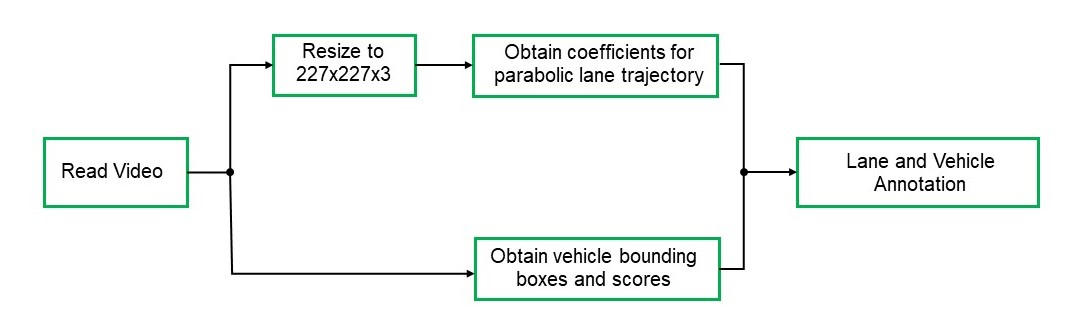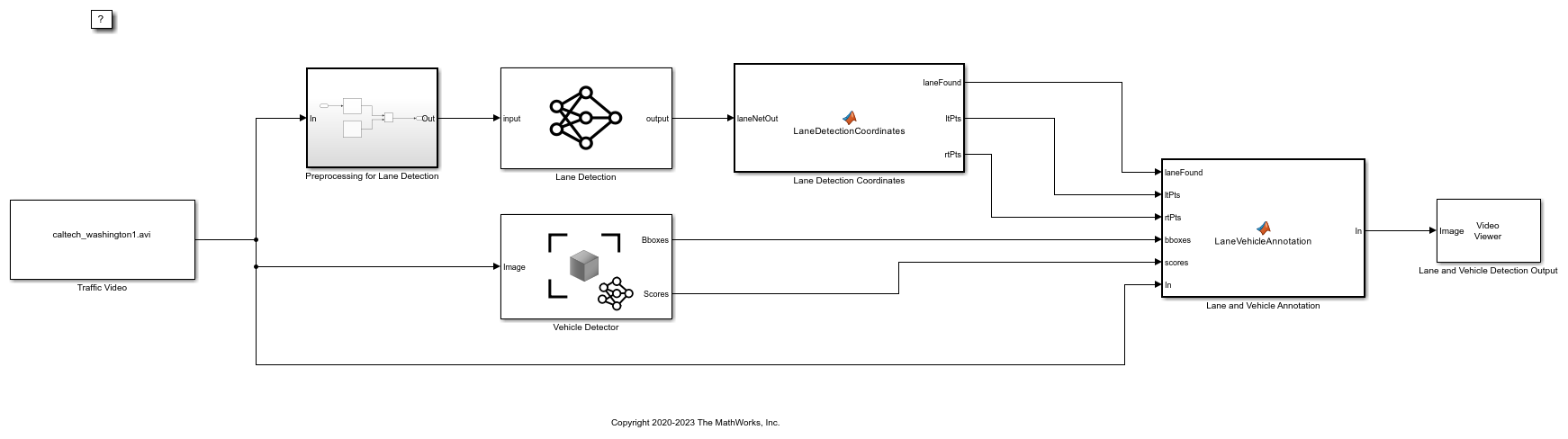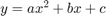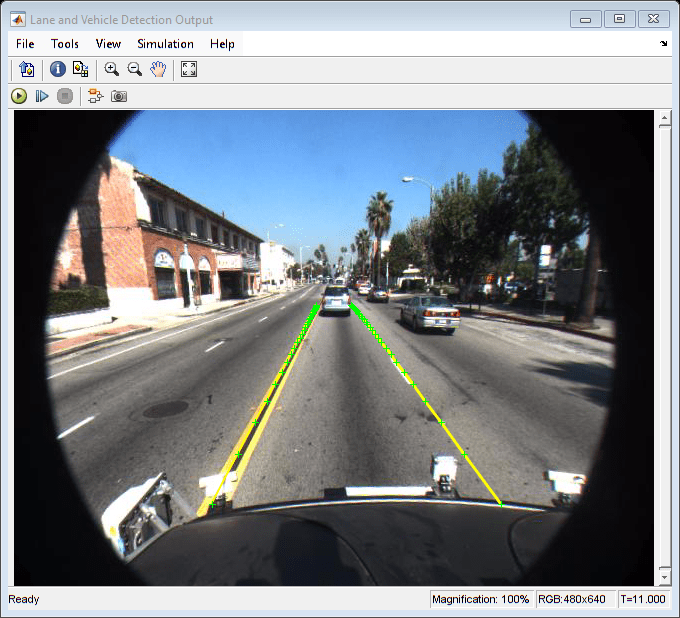Lane and Vehicle Detection in Simulink Using Deep Learning

This example shows how to use deep convolutional neural networks inside a Simulink® model to perform lane and vehicle detection. This example takes the frames from a traffic video as an input, outputs two lane boundaries that correspond to the left and right lanes of the ego vehicle, and detects vehicles in the frame.

This example uses the pretrained lane detection network from the Lane Detection Optimized with GPU Coder example of the GPU Coder Toolbox™. For more information, see Lane Detection Optimized with GPU Coder (GPU Coder).

This example also uses the pretrained vehicle detection network from the Object Detection Using YOLO v2 Deep Learning example of the Computer Vision toolbox™. For more information, see Object Detection Using YOLO v2 Deep Learning (Computer Vision Toolbox).

Algorithmic Workflow

The block diagram for the algorithmic workflow of the Simulink model is shown.Get Pretrained Lane and Vehicle Detection Networks

This example uses the trainedLaneNet and yolov2ResNet50VehicleExample MAT-files containing the pretrained networks. The files are approximately 143MB and 98MB in size, respectively. Download the files from the MathWorks website.

To test the model, the example uses the Caltech lanes dataset. The file is approximately 16 MB in size. Download the files from the MathWorks website.

Lane and Vehicle Detection Simulink Model

The Simulink model for performing lane and vehicle detection on the traffic video is shown. When the model runs, the Video Viewer block displays the traffic video with lane and vehicle annotations.

open_system('laneAndVehicleDetectionMDL');Set the file paths of the dowloaded network model in the predict and detector blocks of the Simulink model. Set the location of the test video to be loaded by the Simulink model.

set_param('laneAndVehicleDetectionMDL/Lane Detection','NetworkFilePath',lanenetFile)
set_param('laneAndVehicleDetectionMDL/Vehicle Detector','DetectorFilePath',vehiclenetFile)
set_param('laneAndVehicleDetectionMDL/Traffic Video','inputFileName',mediaFile)

Lane Detection

For lane detection, the traffic video is preprocessed by resizing each frame of the video to 227-by-227-by-3 and then scaled by a factor of 255. The preprocessed frames are then input to the trainedLaneNet.mat network loaded in the Predict block from the Deep Learning Toolbox™. This network takes an image as an input and outputs two lane boundaries that correspond to the left and right lanes of the ego vehicle. Each lane boundary is represented by the parabolic equation:Here y is the lateral offset and x is the longitudinal distance from the vehicle. The network outputs the three parameters a, b, and c per lane. The network architecture is similar to AlexNet except that the last few layers are replaced by a smaller fully connected layer and regression output layer. The Lane Detection Coordinates MATLAB function block defines a function lane_detection_coordinates that takes the output from the predict block and outputs three parameters; laneFound, ltPts, and rtPts. Thresholding is used to determine if both left and right lane boundaries are both found. If both are found, laneFound is set to be true and the trajectories of the boundaries are calculated and stored in ltPts and rtPts respectively.

type lane_detection_coordinates
function [laneFound,ltPts,rtPts] = lane_detection_coordinates(laneNetOut)

% Copyright 2020-2021 The MathWorks, Inc.

persistent laneCoeffMeans;
if isempty(laneCoeffMeans)
laneCoeffMeans = [-0.0002,0.0002,1.4740,-0.0002,0.0045,-1.3787];
end

persistent laneCoeffStds;
if isempty(laneCoeffStds)
laneCoeffStds = [0.0030,0.0766,0.6313,0.0026,0.0736,0.9846];
end

params = laneNetOut .* laneCoeffStds + laneCoeffMeans;

% 'c' should be more than 0.5 for it to be a right lane
isRightLaneFound = abs(params(6)) > 0.5;
isLeftLaneFound =  abs(params(3)) > 0.5;

persistent vehicleXPoints;
if isempty(vehicleXPoints)
vehicleXPoints = 3:30; %meters, ahead of the sensor
end

ltPts = coder.nullcopy(zeros(28,2,'single'));
rtPts = coder.nullcopy(zeros(28,2,'single'));

if isRightLaneFound && isLeftLaneFound
rtBoundary = params(4:6);
rt_y = computeBoundaryModel(rtBoundary, vehicleXPoints);
ltBoundary = params(1:3);
lt_y = computeBoundaryModel(ltBoundary, vehicleXPoints);

% Visualize lane boundaries of the ego vehicle
tform = get_tformToImage;
% Map vehicle to image coordinates
ltPts =  tform.transformPointsInverse([vehicleXPoints', lt_y']);
rtPts =  tform.transformPointsInverse([vehicleXPoints', rt_y']);
laneFound = true;
else
laneFound = false;
end

end

Vehicle Detection

This example uses a YOLO v2 based network for vehicle detection. A YOLO v2 object detection network is composed of two subnetworks: a feature extraction network followed by a detection network. This pretrained network uses a ResNet-50 for feature extraction. The detection sub-network is a small CNN compared to the feature extraction network and is composed of a few convolutional layers and layers specific to YOLO v2.

The Simulink model performs vehicle detection using the Object Detector block from the Computer Vision Toolbox. This block takes an image as input and outputs the bounding box coordinates along with the confidence scores for vehicles in the image.

Annotation of Vehicle Bounding Boxes and Lane Trajectory in Traffic Video

The Lane and Vehicle Annotation MATLAB function block defines a function lane_vehicle_annotation which annotates the vehicle bounding boxes along with the confidence scores. If laneFound is true, then the left and right lane boundaries stored in ltPts and rtPts are overlayed on the traffic video.

type lane_vehicle_annotation
function In = lane_vehicle_annotation(laneFound,ltPts,rtPts,bboxes,scores,In)

% Copyright 2020-2021 The MathWorks, Inc.

if ~isempty(bboxes)
In = insertObjectAnnotation(In, 'rectangle',bboxes,scores);
end

pts = coder.nullcopy(zeros(28, 4, 'single'));
if laneFound
prevpt =  [ltPts(1,1) ltPts(1,2)];
for k = 2:1:28
pts(k,1:4) = [prevpt ltPts(k,1) ltPts(k,2)];
prevpt = [ltPts(k,1) ltPts(k,2)];
end
In = insertShape(In, 'Line', pts, 'LineWidth', 2);
prevpt =  [rtPts(1,1) rtPts(1,2)];
for k = 2:1:28
pts(k,1:4) = [prevpt rtPts(k,1) rtPts(k,2)];
prevpt = [rtPts(k,1) rtPts(k,2)];
end
In = insertShape(In, 'Line', pts, 'LineWidth', 2);
In = insertMarker(In, ltPts);
In = insertMarker(In, rtPts);
end

end

Run the Simulation

To verify the lane and vehicle detection algorithms and display the lane trajectories, vehicle bounding boxes and scores for the traffic video loaded in the Simulink model, run the simulation.

set_param('laneAndVehicleDetectionMDL', 'SimulationMode', 'Normal');
sim('laneAndVehicleDetectionMDL');On Windows®, the maximum path length of 260 characters can cause "File not found" errors when running the simulation. In such cases, move the example folder to a different location or enable long paths in Windows. For more information, see Maximum Path Length Limitation (Microsoft).

Use Deep Learning Accelerator Libraries

If you have an Intel® CPU that supports AVX2 instructions, you can use the MATLAB Coder Interface for Deep Learning Libraries to accelerate the simulation using Intel MKL-DNN libraries. In the Model Configuration Parameters window, on the Simulation Target pane, set the Language to C++ and the Target library to MKL-DNN.

Code Generation

With GPU Coder, you can accelerate the execution of model on NVIDIA® GPUs and generate CUDA® code for model. For more information, see Code Generation for a Deep Learning Simulink Model that Performs Lane and Vehicle Detection (GPU Coder).